﻿ Grinding Studies of Mango Ginger: Mathematical Modelling of Particle Size Distribution and Energy Consumption

### Grinding Studies of Mango Ginger: Mathematical Modelling of Particle Size Distribution and Energy Co...

Thirupathihalli Pandurangappa Krishna Murthy, Balaraman ManoharOPEN ACCESSPEER-REVIEWED

## Grinding Studies of Mango Ginger: Mathematical Modelling of Particle Size Distribution and Energy Consumption

Thirupathihalli Pandurangappa Krishna Murthy1,, Balaraman Manohar2

1Department of Biotechnology, Sapthagiri College of Engineering, Bangalore, India

2Department of Food Engineering, CSIR-Central Food Technological Research Institute, Mysore, India

### Abstract

Mango ginger was ground in hammer mill with three different classifying screens and pin mill to study the particle size distribution and energy consumption. The Rosin-Rammler Bennet (RRB) model fitted well the particle size distribution data over the entire range of the size distribution for grinding in both hammer mill and pin mill with high coefficient of determination (R2) and low values of residual sum square, root mean square error and Chi-square. Relationship between RRB model parameters with hammer mill screen size was obtained with high R2. All the three classical models such as Rittinger’s, Kick’s and Bond’s law were found suitable to explain the energy consumption for grinding. Energy consumption increased exponentially with decrease in classifying screen size of hammer mill. The Work index for grinding increased with increase in size reduction ratios and were in the range of 0.075-0.58 kW/kg.

### At a glance: Figures

1
Prev Next

• Murthy, Thirupathihalli Pandurangappa Krishna, and Balaraman Manohar. "Grinding Studies of Mango Ginger: Mathematical Modelling of Particle Size Distribution and Energy Consumption." American Journal of Food Science and Technology 1.4 (2013): 70-76.
• Murthy, T. P. K. , & Manohar, B. (2013). Grinding Studies of Mango Ginger: Mathematical Modelling of Particle Size Distribution and Energy Consumption. American Journal of Food Science and Technology, 1(4), 70-76.
• Murthy, Thirupathihalli Pandurangappa Krishna, and Balaraman Manohar. "Grinding Studies of Mango Ginger: Mathematical Modelling of Particle Size Distribution and Energy Consumption." American Journal of Food Science and Technology 1, no. 4 (2013): 70-76.

 Import into BibTeX Import into EndNote Import into RefMan Import into RefWorks

### 1. Introduction

Curcuma amada popularly known as mango ginger is a perennial, rhizomatous herb and unique species belonging to Zingiberace family resembles Zinger but imparts raw mango flavor. C.amada originated in the Indo-Malayan region is widely distributed and cultivated in different parts of India. Due to its exotic flavor of raw unripe mango it is used in pickles, candies, curries, salads, etc. in Indian subcontinent [1, 2]. The volatile oils of the mango ginger contain the mixture of compounds present in both raw mango and turmeric [3, 4]. The spice is credited with applications in traditional Ayurveda and Unani medicine system as appetizer, antipyretic, laxative, diuretic, emollient etc. It is also having biological properties like antioxidant [5, 6], antimicrobial , antifungal , anti-inflammatory  activity etc. Mango ginger is also an unconventional source of starch having potential functional properties .

Size reduction or communition is an important unit operation that changes the particle size and shape, increases the bulk density, improves the flow properties, increases porosity and generates new surface area. However physical and flow properties of biological material are highly dependent on particle size and distribution [11, 12]. The extraction of naturaceuticals from plant material requires the pre-processing of the extraction material in order to reduce the particle size. Increased surface increases the contact points for the extraction of the bioactive compounds thereby improving the efficiency of extraction.

Several empirical models have long been used to describe the Particle size distribution (PSD) of powders. Most commonly used distribution functions are Rosin-Rammer (RR), Gaudin-Schuhmann (GS) and log-normal. Out of these various models, RR equation is reportedly found fitting the best to the experimental size data for wide range of materials [13, 14, 15].

Grinding is a very inefficient process and it is important to use energy as efficiently as possible. Mechanical energy is required to breakdown the materials and also to overcome the friction between the moving parts of the machine. Almost all of the energy in the grinding process is wasted as heat and only 0.06 - 1% of the input energy is utilized for the size reduction of the material [16, 17]. The energy consumption of grinding material depends on the reduction ratio, moisture content, bulk density, feed rate of the material and machine variables . The energy required to obtain small particle size is relatively high. Classification screen size was the most significant factor affecting the performance of the hammer mill . Unfortunately, it is not easy to calculate the minimum energy required for a given reduction process, but some theories have been advanced which are useful. Models such as Kick, Rittinger and Bond have been used by many researchers to predict the energy consumption during the grinding of agricultural material . Effect of various types of milling equipment such as attrition, abrasion, pin and hammer mill on physic-chemical properties of finger millet have been studied . Several studies have been found on energy for grinding of wheat , carrot , gum karaya , maize , pepper , coconut , turmeric  and cumin . Extensive analysis of PSD of turmeric powder obtained in conventional and cryogenic grinding process has been dealt .

The aims of the present investigation are: a) mathematical modeling of the particle size distribution of mango ginger powder ground in hammer mill with different classifying screen sizes and pin mill using different mathematical models and b) to relate energy consumption for communition with the particle size using various energy laws.

### 2. Materials and Method

2.1. Material

Fresh mango ginger rhizomes were procured from the local market, Mysore. Rhizome were washed to remove dirt and sliced using a vegetable slicer (M/s Robot coupe, USA, Model: CL 50 Gourmet). Sliced mango ginger was dried in a hot air tray drier (M/s Technico Laboratory Products, Chennai) dryer 40 ˚C till the moisture level reduces to 8%. The dried material is used further in grinding studies. Moisture content was estimated by toluene distillation method as per ASTA.

2.2. Grinding Experiment

Dried mango ginger was ground in impact type hammer mill (model: CMC/CM- Q/753/97, M/s Cadmach Machinery Company Pvt. Ltd, Ahmedabad, India) with three different classifying screens (AP125, QS36, QS31 having screen openings 3 mm, 1.6 mm 0.5 mm respectively) and pin mill (model: 160 UPZ, M/S Alpine, Germany) at a feed rate of 5.7 kg/hr. Energy meter (MilestoneTM LD-15U , MilestoneTM Electronics Pvt. Ltd., India) was used to measure the energy consumption during the process of grinding. The energy reported in our studies is the net energy for grinding after deducting the energy required for running the equipment with no loads.

2.3. Sieve Analysis

Ground mango ginger powder was separated into different particle size fractions using a set of sieves in a laboratory sieve shaker (M/s Muhlenbau, Germany). The set of standard sieves was arranged serially in a stack with smallest mesh sieve at bottom and the largest at the top. About 100g of ground mango ginger powder was loaded on the top screen and the stack was shaken for 15 minutes. The material retained on each screen was removed, weighed, and the mass fraction also determined. Three set of experiments were carried out and average values were reported.

2.4. Mathematical Function for Particle Size Distribution

Particle size distribution data of mango ginger powder obtained by grinding in hammer mill and pin mill are represented by mathematical functions. The mathematical functions used to describe the size distribution data of PSD of powders of vary types and sizes are presented as follows:

(1) Rosin-Rammler-Bennett (RRB) equation [29, 30, 31]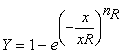(1)

(2) Gaudin-Schuhmann (GS) equation [31, 32]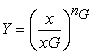(2)

Where Y is the cumulative mass fraction (%), x is the particle size, xR, xG are size parameter of RRB and GS model respectively, nR, nG are distribution parameter of RRB and GS model respectively.

(3) Log-normal distribution

Another function which has been in wide use for the analysis of comminution is the log-normal distribution function  which is as below: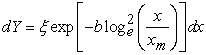(3)

where b is the steepness constant =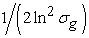,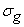is the size ratio corresponding to the 84% cumulative undersize mass fraction (x84) and the 50% cumulative undersize mass fraction (x50).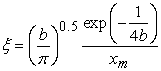(4)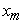represents the mode of the distribution which is equal to c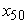.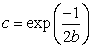(5)
2.5. Mathematical Function for Grinding Energy Calculation

After carrying out sieve analysis of the powder, the final particle size (L2) was evaluated by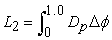(6)

Where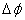is the weight fraction of particles of diameter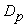, whereis the average of the aperture sizes of the sieves [23, 34].

The size reduction is quantified by comparing the new surface area generated to the energy consumed for generating that area. Mathematically, it is expressed as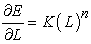(7)

Where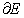is the differential energy required to produce a change,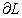, in a particle of typical size dimension, L, and K and n are constants [34, 35]. For Kick, Rettinger and Bond models, values of n were assuemed as -1, -2, -3/2, respectively and the following expressions derived: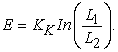(8)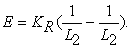(9)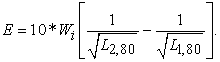(10)

Where L1 and L2 and L1,80 and L2,80 are initial and final particle size of mango ginger at 100 and 80% of cumulative weight fraction.

The greatest use of these equations is in making comparisons between power requirements for various degrees of reduction .

2.6. Size parameters of the Distribution

From the graph of cumulative weight fraction vs particle size (Figure 1), one can obtain particle size xi corresponding to any cumulative weight % (Yi) where ‘i’ denotes percentage value. For example, x80 is the value of particle size in x-axis of the graph corresponding to Y value of 80% for a particular grinding setup. To analyze the distribution width, Mass relative span used as an indicator and can be calculated using Eq.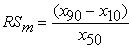(11)

RSm provides a dimensionless measure of particle size distribution . x90, x50, x10 are the particle size at 10, 50 and 90 % of cumulative mass fraction. x50 and x10 is also known as media length and effective size respectively .

Figure 1. Particle size distribution of mango ginger powder

Skewness is another important characteristic among the PSD. It measures degree of asymmetry of normal distribution curve and its sign denotes whether a curve has an asymmetrical tail to its left of right when distribution is plotted versus particle size. Inclusive graphic skewness of particle distribution which includes 90% of the curve (Folk, 1974) was calculated from the Eq.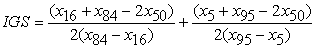(12)

Where IGS is Inclusive graphic skewness and x5, x16, x84 and x95 are particle sizes in µm corresponding to 5, 16, 84 and 95 % of cumulative mass fraction. The interval between x5 and x95 points on normal probability curve should be exactly 2.44 times the interval between x25 and x75 points. It represents the departure from the above ratio or normality. Kurtosis measures the sorting in central portion. The kurtosis of PSD which includes 90% of the curve is presented in Eq.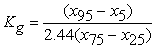(13)

Where Kg is the graphic kurtosis, x25 and x75 are the particle sizes corresponding to 25 and75% cumulative undersize mass fraction respectively .

Canadian Fertilizer Institute  procedure was used generally to determine uniformity index and size guide number. Due to some limitations modified relations were used in this study as described by .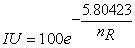(14)

Where IU is the uniformity index (%) and nR is the rosin rammer distribution parameter.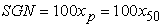(15)

Where SGN is the size guide number (dimensionless), xp is the particle size in µm and x50 is the median length µm. Substituting F(x) =50 and xp=x50 in Eq.15, the median length was derived by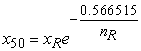(16)

Where xR and nR are Rosin-Rammler size parameter and distribution parameter respectively.

Then Eq. 15 becomes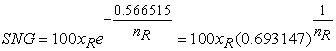(17)

The coefficient of uniformity and the coefficient of graduation of the particle size distribution were evaluated as follows .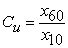(18)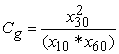(19)

Where Cu and Cg are the coefficient of uniformity and coefficient of gradation, they are dimensionless numbers. x10 is the effective size, µm and x30 and x60 are the particle sizes in µm corresponding to the 30 and 60% cumulative undersize masses respectively.

Distribution of standard geometric deviation of high region (GSD1- between x16 and x50) and the Distribution of standard geometric deviation of low region (GSD2-between x84 and x16) and the Distribution of standard geometric deviation of the total region (GSD12-between x84 and x16) was determined as follows .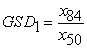(20)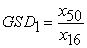(21)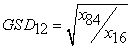(22)

Where x16, x50 and x84 are the particle size in µm corresponding to 16, 50 and 84% cummulative undersize mass fraction respectively.

xR, x50, x10, SGN are called size related parameters whereasnR, RSm, IU, Cu, Cg and GSD are called distribution related parameters.

2.7. Statistical Analysis

Nonlinear Least square method using the SOLVER tool based on the Generalized Reduced Gradient (GRG) method of iteration available in Microsoft Excel (Microsoft Office 2010, USA) was used to fit the experimental data to selected models. For evaluating the goodness of fit, four statistical parameters such as residual sum square (RSS), root mean square error (RMSE), chi square (CS) were used in addition to coefficient of determination (R2) as primary criterion. The values of R2 were one of the primary criterions for selecting the best model and can be used to test linear relationship between experimental and model predicted values.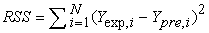(23)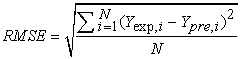(24)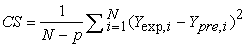(25)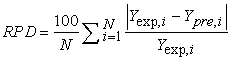(26)

Where N is the total number of observations, p is number of factors in the mathematical model, Yexp,i and Ypre,i are the experimental and predicted cumulative mass fraction at any observation i.

### 3. Results and Discussion

3.1. Effect of Grinding Conditions on Particle Size Distribution (PSD)

The values of weights of the different particle size obtained in the sieving operations are converted to the mass fraction and cumulative mass fractions were obtained for each experimental runs. The values of cumulative weight fraction were regressed against the sieve size to selected mathematical models (Section 2.4) to describe the particle size distribution. The statistical values and model parameters were presented in Table 1 and Table 2. RRB equation was selected model which fit best to the experimental data with higher R2 and lower value of RSS, chi-square and RMSE (Figure 1). The distribution parameter (nR) values decreased with decrease in hammer mill screen size indicating decreasing of uniformity of particle size distribution as screen size decreased in hammer mill. In pin mill also uniformity index is having higher values. The size parameter values of RRB equation also decreased with decrease in screen size.

#### Table 2. Numerical values of log-normal distribution parameters and statistical values

The correlation as developed between the hammer mill screen size and RRB mathematical function parameters. The screen size related distribution parameter using logarithmic equation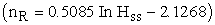which gave the best fit for the experimental data with R2 of 0.9946. Another correlation has developed between hammer mill sieve size and size parameter and correlated with power law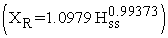which gave best fit with the experimental data with R2 of 0.993. Where yss is the hammer mill screen size, nR and xR is RRB models distribution parameter and sieve parameter respectively. Average particle size the ground sample decreased with decrease in hammer sieve size and also in pin mill average particle size decreased. The power law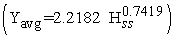describes the relation between average particle size and the hammer mill sieve size with correlation of determination of 0.999. Where Yavg is average particle size and Hssis hammer mill sieve size.

3.2. Effect of Grinding Conditions on Energy Consumption

Energy consumed during different grinding conditions was calculated using Eq.27 and expressed in kJ/kg.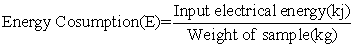(27)

Energy consumption is directly proportional to hammer mill sieve size. As the sieve size of hammer mill decreased, the grinding energy consumption increased rapidly. Power consumption in pin mill is much higher compared to hammer mill. There is a strong relationship between hammer mill sieve size and energy consumption. An exponential model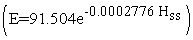fits best to the experimental data with R2 value of 0.999.Where E is the energy consumed during grinding process in kJ/kg and Hss is hammer mill screen size in µm.

Reduction ratio is a dimensionless number indicates the ratio between initial particle sizes to final particle size. It explains the degree of reduction of particle size in feed and ground material. It was calculated as follows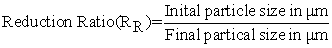(28)

Reduction ratio is inversely proportional to the hammer mill sieve size. Due to decrease in sieve size, the reduction increased rapidly. This shows the extent of grinding and by using lower sieve size in hammer mill finest particle can obtain. In the reduction ratio is very high compared to hammer mill because average particle size is very less. Another correlation has developed between hammer mill sieve size and size parameter and correlated with power law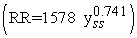which gave best fit with the experimental data with R2 of 0.999. The energy consumption logarithmically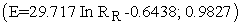increases with increase in reduction ratio. Where E is the energy consumption in kJ/kg and RR is reduction ratio.

Specific energy consumption is the ratio energy consumed during to reduction ratio obtained at that grinding condition. It was calculated using following expression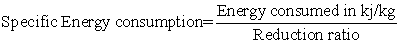(29)

Specific energy consumption was decreased with decrease in hammer mill sieve size. In pin mill it is slighter higher than the smaller sieve size of the hammer mill. Specific energy values are presented in Table 3.

Other three classical models viz, Rittinger’s, Kick’s and Bonds law selected to relate the energy consumption to particle size also gave reasonably good results. All the numerical values for Rittinger constant, kicks constant and Bonds work index was presented in Table 3. Work index increased with increase in hammer mill sieve size and it is very high for the pin mill. In general more energy is required to grinding smaller particles. The work index also increases logarithmically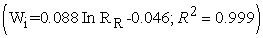with increase in hammer mill sieve size. The work index values of present work were compared with the previous literature available for grinding studies of different food materials (Table 3).

#### Table 3. Constant values of various energy laws with reduction ratio, specific energy consumption and work index

3.3. Data analysis of Particle Size Distribution

The entire sieve related and distribution related parameters were calculated using the equations presented in section 2.6. The median length, effective size, RRB sieve parameter and Size guide number are size related parameters. The median length increases with increase in hammer mill sieve size due to fine skewness of the distribution. Same trend was followed by effective size. The size guide number also directly proportional to the hammer mill sieve size and values increased with increase in hammer mill sieve size. The median length, effective size and size guide number values of in mill are less compared to hammer mill due to the less particle size of the ground range. There was a strong correlation between hammer mill sieve size and size parameters and successfully predicted by the logarithmic equation with high coefficient of determination values. The numerical values with R2 are presented in Table

nR of RRB equation, relative mass span, uniformity index, coefficient of uniformity, coefficient of gradation and geometric standard deviation are distribution related parameters. The graphic skewness and kurtosis decreased with increase in sieve size of the hammer mill. The uniformity index increased with increase in the hammer mill sieve size due to decrease in relative mass span and skewness as screen size increased. Coefficient of uniformity and coefficient of gradation also decrease with increase in hammer mill sieve size. Coefficient of uniformity is more than 4 indicate a wide range of distribution and well graded particle size distribution. Coefficient of gradation range between 1 to 3 shows well graded particles. But the values are little out of range. The Distribution geometric standard deviation of high range and low range also decreased with increase in the hammer mill sieve size. The Distribution geometric standard deviation for total region also followed the same trend. The correlation was developed between Hammer mill sieve size and the distribution parameter. Logarithmic equation explains the relation best with the higher value of R2. The regression coefficients along with the parameter values are presented in Table 4.

### 4. Conclusion

Particle size is the important single physical characteristics of solid which is necessary to determine the correct particle size distribution, prior to utilization in extraction. The Rosin Rammer Bennet equation produces reasonably good fit of Particle size distribution over entire range of cumulative weight fraction with high values of coefficient of determination. On the other hand Gudin Schuman equation and Log normal distribution does not properly fit the experimental data. Energy consumption increased with the hammer mill decrease in hammer mill sieve size. Reduction ratio is very high at lower hammer mill sieve size.

### References

  Sasikumar, B, 2005. Genetic resources of curcuma: diversity, characterization and utilization. Plant Gen. Res., 3: 230-251.In article CrossRef  Policegoudra, R.S., S.M. Aradhya and L. Singh, 2001. Mango ginger (Curcuma amada Roxb.)-A promising spice for photochemical and biological activities. J. Biosci., 36: 739-748.In article CrossRef  Golap, S. G and C. Bandyopadhyaya, 1984. Characterization of mango-like aroma in Curcuma amada Roxb. J. Agric. Food Chem., 32: 57-59.In article CrossRef  Rao, A. S., B. Rajanikanth and R. Seshadri, 1989. Volatile aroma components of Curcuma amada Roxb. J. Agric. Food Chem., 37: 740-743.In article CrossRef  Prakash, D., S. Suri., G. Upadhyay and B. Singh, 2007. Total phenol, antioxidant and free radical scavenging activities of some medicinal plants. Food Sci. Nutri. Int. J., 58: 18-28.In article CrossRef  Policegoudra, R.S., K. Abiraj., D. Channe Gowda and S. M. Aradhya, 2007. Isolation and characterization of antioxidant and antibacterial compound from mango ginger (Curcuma amada Roxb.) rhizome. J. Chrom. B., 852: 40-48.In article CrossRef PubMed  Policegoudra, R. S., S. Divakar and S. M. Aradhya, 2007. Identification of Difurocumenonol, a new antimicrobial compound isolated from mango ginger (Curcuma amada Roxb.) rhizome. J. Appl. Micro., 102: 1594-1602.In article CrossRef PubMed  Singh, G., O. P. Singh and S. Maurya, 2002. Chemical and biocidal investigations on essential oils of some Indian Curcuma species. Prog. Crystal Growth Charac., 45: 75-81.In article CrossRef  Mujumdar, A. M., D. G. Naik., C.N. Dandge and H. M. Puntambekar, 2000. Anti-inflammatory activity of Curcuma amada Roxb. in albino rats. Ind. J. Pharm., 32: 375-377.In article  Policegoudra, R. S and S. M. Aradhya, 2008. Structure and biochemical properties of starch from an unconventional source - a mango ginger (Curcuma amada Roxb.) rhizome. J. Food Hydro., 22: 513-519.In article CrossRef  Dryzmala, Z. 1993. Industrial briquetting-fundamentals and methods. Stud. Mech. Engine., PWN-Polish Scientific Publishers, Waraszawa, Pp. 13.In article  Baitra, V. S. P., A. R. Womac., N. Chevanan., P. I. Miu., I. Igathinathane., S. Sokhansanj and D. R. Smith, 2009. Direct mechanical energy measures of hammer mill comminution of switch grass, wheat straw, and corn stover and analysis of their particle size distributions. Powder Technol., 193: 32-45.In article CrossRef  Manohar, B and B. S. Sridhar, 2001. Size and shape characterization of conventionally ground turmeric (Curcuma domestica) particles. Powder Technol., 120: 292-297.In article CrossRef  Baitra, V. S. P., A. R. Womac., Y. T. Yang., P. I. Miu., I. Igathinathane and S.Sokhansanj, 2009b. Mathematical model parameters for describing the particle size spectra of knife-milled corn stover. Biosyst. Engin, 104: 369-383.In article  Macias-Garcia, A., M. Cuerda-Correa and M. A. Diaz-Diez, 2004. Application of Rosin-Rammler and Gauding-Schuhmann models to the particle size distribution analysis of agglomerated cork. Materials Char., 52: 159-164.In article CrossRef  Ghorbani, Z. A., A. Masoumi and A. Hemmat, 2010. Specific energy consumption for reducing the size of alfalfa chops using a hammer mill. Biosyst. Engin., 105: 34-40.In article  Mohsenin, N. N, 1986. Physical properties of plant and animal materials. Gordon and Breach Science publishers, New York.In article  Lopo, P, 2002. The right grinding solution for you: roll, horizontal or vertical. Food Manag., 53: 23-26.In article  Fang, Q., I. Boloni., E. Haque and G. K. Spillman, 1997. Comparison of energy efficiency between a roller mill and a hammer mill. Trans. Amer. Soci. Agric. Engin., 13: 631-635.In article  Fellows, P. J, 2000. Food processing Technology-Principles and Practice. Woodhead Publishing, Cambridge, pp.13-14.In article CrossRef  Katti, S.V., S. Kumar and N. G. Mallesh, 2008. Studies on the effect of milling finger millet in different pulverisers on physic-chemical properties of the flour. J. Food Sci. Technol., 45: 398-405.In article  S. G. Walde, K. Balaswamy, V. Velu and D. G. Rao, 2002. Microwave drying characteristics of wheat. J. Food Engin., 55: 271-276.In article CrossRef  A. Cakkaravarthi., R. G. Math., S. G. Walde and D. G. Rao, 1993. Grinding characteristics of carrots (Dacus Carota L.). J. Food Engin., 20: 381-389.In article CrossRef  Walde, S. G., K. Balaswamy., R. Shivaswamy., A. Chakkaravarthi and D. G. Rao, 1997. Microwave drying and grinding characteristics of gum karaya (sterculia urens). J. Food Engin., 31: 305-313.In article CrossRef  Velu, V., A. Nagendrer., P. G. P. Rao and D. G. Rao, 2006. Dry milling characteristics of microwave dried maize grains. J. Food Engin., 74: 30-36.In article CrossRef  Murthy, C. T. 2001. Cryogenic Size reduction and Engineering properties of black pepper,” PhD thesis, University of Mysore, Mysore.In article  Ragavendra , S.N., S.R. Ramachandra Swamy., N. K. Rastogi., K. S. M. S. Raghavarao., S. Kumar and R. N. Tharanathan, 2006. Grinding characteristics and hydration properties of coconut residue: A source of dietary fiber. J. Food Engin., 72: 281-286.In article CrossRef  Goswami and Manish Singh, 2003. Role of feed rate and temperature in attrition grinding of cumin. J. Food Engin., 59: 285-290.In article CrossRef  Rosin, P and E. Rammler , 1933.The laws governing the fineness of powdered coal. J. Inst. Fuel., 7: 29-36.In article  Bennette, J. G, 1936. Broken coal. J. Inst. Fuel., 10: 22-39.In article  Harris, C.C. 1968. The application of size distribution equation to multi-event communition processes. Trans. SME/AIME., 241: 343-358.In article  Schuhmann, R. 1940. American Institute of Mining and Metallurgical Engineers. Technical publication, New York., pp.1189.In article  Prasher, P.L. 1987. Crushing and Grinding Process Handbook. Wiley, New York.In article  Earle. R.L. Unit operations in Food Processing. Pergamon, Oxford.In article  Sharma, P., A. Chakkaravarthi., V. Singh and R. Subramanian, 2008. Grinding Characteristics and batter quality of rice in different wet grinding systems. J. Food Engin., 88: 499-506.In article CrossRef  Allais, I., R. Edoura-Gaena., J. Gros and G. Trystram, 2006. Influence of egg type, pressure and mode of incorporation on density and bubble distribution of a lady finger batter. J. Food Engin., 74: 198-210.In article CrossRef  Craig, R.F, 2004. Craig's Soil Mechanics. Spon Press, London.In article  Folk, R. L, 1974. Petrology of Sedimentary Rocks. Austin, TX : Hemphil Publishing Co.In article  CFI, 1982. The CFI Guide of Material Selection for the Production of Quality Blends. Canadian Fertilizer Institute, Ottawa, Ontario, Canada.In article  Perfect, E and Q. Xu, 1998. Improved parameterization of fertilizer particle size distribution. AOAC Int. J., 81: 935-942.In article  Hinds, W. C, 1992. Properties, Behaviour and Measurement of Airborne Particles. Aerosol Technology- John Wiely and Sons, New York.In article